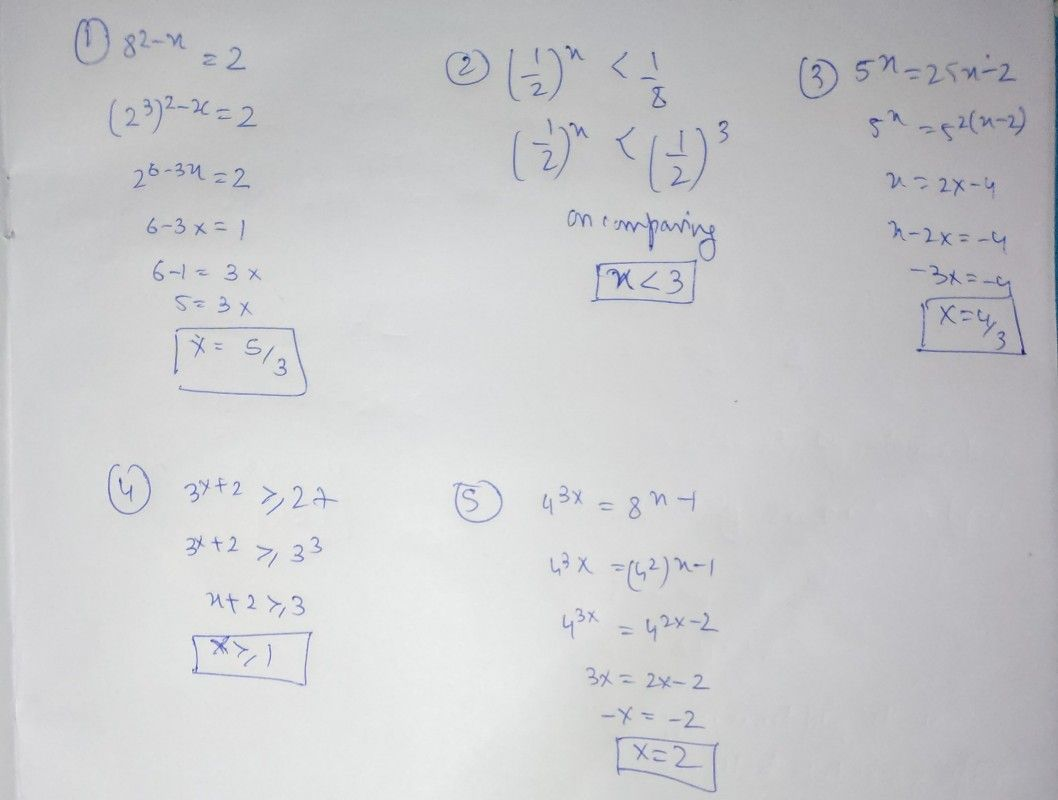Symbol
Problem$xcct1on3$ Solve for the values of x for each of the following exponential equations $254$ inequalities. $1$ $82-x=2$ $2$ $\left(1/2\right)x<1/8$ $5x=25x-2$ $3$ $4$ $3x+2\geq 27$ $543y$ $43y=Bx-1$
10th-13th grade
Other
Search count: 105
SolutionQanda teacher - RakeshRajpls. rate it thanks